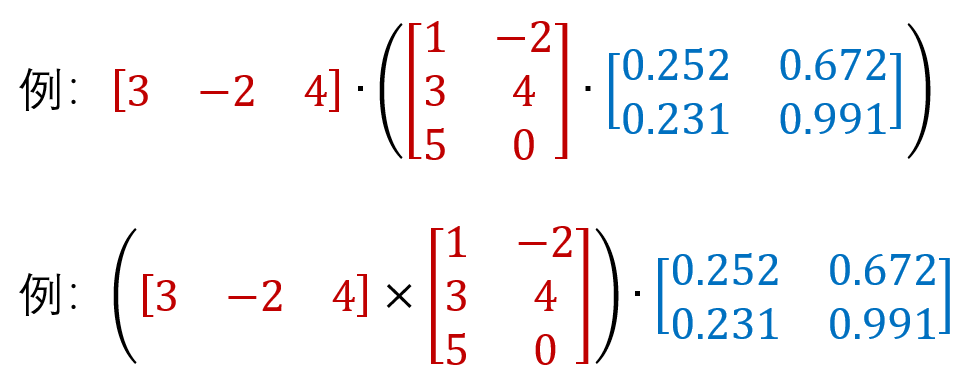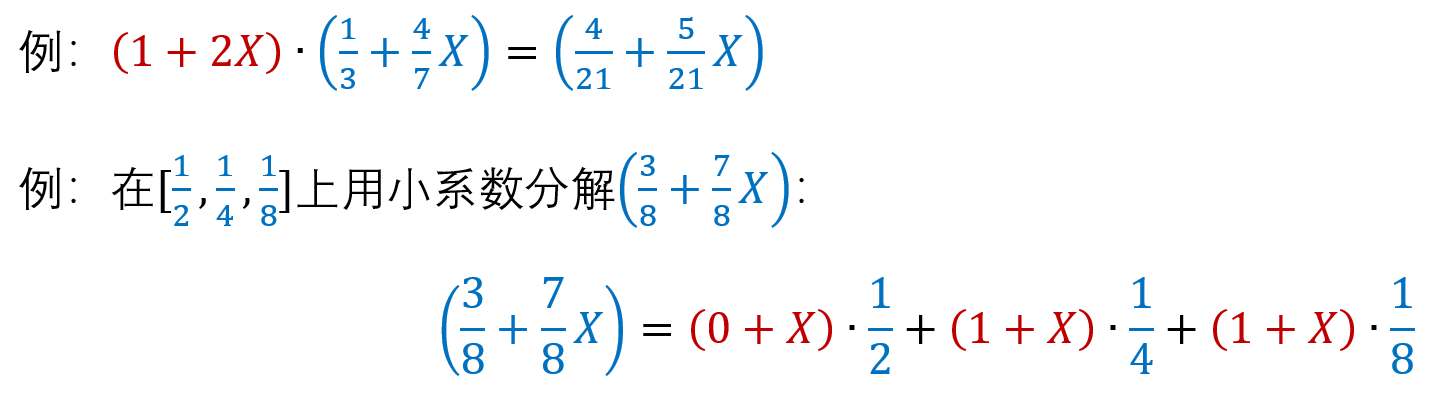08/12 12:17

# 全同态加密## 全同态算法应用之一：外包计算。## TFHE与全同态## 全同态加密中的噪声1. LHE 层级全同态
通过设置LHE中的安全参数，使之足够大从而支持一定深度(depth)的布尔电路的评估。
想象上图中最左端的密文全都有3个level，密文每通过一个 与/或门 就会消耗一个level(取非操作相比于 与/或门 产生可忽略的噪声)，如果最终的level小于0则密文不能够正确解密，如下图所示：2. Bootstrapping 自举
2009年Gentry提出了自举的最naïve的设想。整个自举过程如下：1.现有一明文m。
2.使用 p k 1 pk_1 pk1对该明文进行加密，得到一个密文，此时密文新鲜(fresh)，噪声较小。假设如果噪声不超过框中的红线，就可以正确地进行解密。
3.经过一系列的评估操作之后，噪声不断增大，现在噪声已经达到红线，必须将噪声减小才能继续进行计算。最直观地方法就是对其进行解密，因为解密之后噪声会消失，但是解密需要密钥，如果这个过程在云服务器上执行的话客户端是不可能给出自己的私钥的。
4.于是Gentry想了一个办法，他将解密函数Dec作为评估函数中的f，再用一个新的公钥 p k 2 pk_2 pk2将整个密文加密，用户的私钥 s k 1 sk_1 sk1(对应公钥 p k 1 pk_1 pk1)也使用 p k 2 pk_2 pk2进行加密后给云服务器。
5.这时候云服务器就可以在 p k 2 pk_2 pk2加密下进行同态解密，从而得到 p k 2 pk_2 pk2加密的f(m)，此时噪声比之前少了许多，能够支持至少一次的乘法运算。
通过这样的自举步骤，同态加密的运算便能够不断地持续地进行。

LHE 和 Bootstrapping 两种方案各有优缺点：

TFHE在 LHE 方案和 Bootstrapping 方案中都实现了一定的改进，接下来详细介绍TFHE。

# TFHE

## Torus 环面

TFHE全称为：Fully Homomorphic Encryption over the Torus。FHE代表全同态加密，而T代表环面(Torus)。因此，想了解TFHE，在了解什么是FHE后，还要了解什么是环面。1. 它是一个阿贝尔群(环面上的加法是有定义的)。
2. 它拥有 Z {\color{red}\mathbb Z} Z-module 结构。
3. 它不是一个环(环面上的元素之间的乘法没有定义)。## TLWE密文的对称加密

1. 假设消息空间 M = { 0 , 1 / 3 , 2 / 3 } M=\{0,1/3,2/3\} M={0,1/3,2/3} 是实数环面上逆时针三元消息空间。
2. 假设现在要加密明文消息 μ = 1 / 3 m o d 1 ∈ M \mu=1/3\quad mod \quad 1 \in M μ=1/3mod1M
3. 现在给消息添加一个高斯噪声：计算phase： φ = μ + G a u s s i a n e r r o r \varphi = \mu + Gaussian error φ=μ+Gaussianerror
4. 然后在环面上随机选择一个 a a a作为mask(当 a = 0 a=0 a=0时为平凡(trivial)的情况，此时明文与密钥相互独立，没有加密的效果)。5. 随后可以使用密钥s进行加密，得到密文： b = s ⋅ a + φ b=s\sdot a+\varphi b=sa+φ(LWE形式，安全性基于LWE问题的困难性)。加密后的密文 b b b看起来像一个随机数。
6. 同样也可以使用密钥s进行解密，得到phase： φ = b − s ⋅ a \varphi=b-s\sdot a φ=bsa，再对phase进行取整(round)到最近的消息空间中的元素(也可以求期望)，最终可得到消息 μ \mu μTLWE密文具有同态性质，在密文上进行线性组合，其对应明文也会做相应的线性组合，噪声也随之增加。换句话说，我们对TLWE上的元素做一个线性组合，那它们的 a a a b b b也会有相应的线性关系，如果我们把它解密， φ \varphi φ也会有同样的关系。因为我们添加的噪声是高斯噪声，我们对 φ \varphi φ求平均得到消息 μ \mu μ，也会满足这个关系。这是环面上自带的加同态的性质，对于全同态加密的设计来说是一个很好的性质。## TFHE的三种密文形式

• TLWE 密文： c = ( a , b ) ∈ T n + 1 c=(a,b)\in \mathbb T^{n+1} c=(a,b)Tn+1
其中 b = s ⋅ a + φ , φ = e + μ b=s\sdot a+\varphi,\varphi=e+\mu b=sa+φ,φ=e+μ
• TRLWE 密文： c = ( a , b ) ∈ T N [ X ] 2 c=(a,b)\in \mathbb T_N[X]^2 c=(a,b)TN[X]2
其中 b = s ⋅ a + e + μ , e ∈ T N [ X ] G a u s s i a n b=s\sdot a+e+\mu,e \in \mathbb T_N[X]Gaussian b=sa+e+μ,eTN[X]Gaussian
• TRGSW 密文： C = Z + m ⋅ G 2 ∈ T n [ X ] 2 l × 2 C=Z+m\sdot G_2\in \mathbb T_n[X]^{2 l \times 2} C=Z+mG2Tn[X]2l×2
其中 Z Z Z 为长 2 l 2l 2l 的一列0的RLWE加密， G 2 G_2 G2为 gadget 矩阵。TLWE T \mathbb T T T n + 1 \mathbb T^{n+1} Tn+1 √ \surd × \times × B n \mathbb B^n Bn
TRLWE T N [ X ] \mathbb T_N[X] TN[X] T N [ X ] 2 \mathbb T_N[X]^2 TN[X]2 √ \surd × \times × B N [ X ] \mathbb B_N[X] BN[X]
TRGSW Z [ X ] / ( X n + 1 ) \mathbb Z[X]/(X^n+1) Z[X]/(Xn+1) T N [ X ] 2 l × 2 \mathbb T_N[X]^{2l\times 2} TN[X]2l×2 √ \surd √ \surd B N [ X ] \mathbb B_N[X] BN[X]

## TFHE的外积加速# 层次TFHE(TFHE IN LEVELED MODE)1. Or, And, Not
DNF logic
2. Xor, And, 1
Multivariate polynomials
3. Nand
minimal indeed
4. Mux, 0, 1

Mux门电路的选择逻辑很简单，如果c为0则输出b，如果c为1则输出a：
C M u x ( c , a , b ) = b + c ⊡ ( a − b ) CMux(c,a,b)=b+c\boxdot(a-b) CMux(c,a,b)=b+c(ab)# 全同态TFHE(TFHE IN FHE MODE)1. 首先从一个(平凡的)TLWE ( v 0 + v 1 X + … + v ( N − 1 ) X ( N − 1 ) ) a (v_0+v_1X+…+v_(N-1)X^(N-1))^a (v0+v1X++v(N1)X(N1))a开始
2. 将其旋转 p = − φ s ( a , b ) p=-\varphi_s(a,b) p=φs(a,b)个位置
3. 取出常数项constant term(其加密 v p v_p vp)p p p已知的情况下，旋转操作为 ( X p ⋅ c ) (X^p \cdot c) (Xpc)
p p p未知的情况下，旋转操作为 ( T G S W ( X p ) ⊡ c ) (TGSW(X^p) \boxdot c) (TGSW(Xp)c)

1. 首先反方向旋转 b b b个位置，即Multiply by X − b X^{-b} Xb b b b为密文，事先知道可以直接计算。
2. For i ∈ [ 1 , n ] i \in [1,n] i[1,n] multiply by T G S W ( X − a i s i ) TGSW(X^{-a_i{\color{red}s_i}}) TGSW(Xaisi)
X a i s i = 1 + ( X a i − 1 ) ⋅ s i , s i ∈ { 0 , 1 } X^{a_i{\color{red}s_i}}=1+(X^{a_i}-1)\sdot{\color{red}s_i},{\color{red}s_i}\in\{0,1\} Xaisi=1+(Xai1)si,si{0,1}
T G S W ( X a i s i ) = h + ( X a i − 1 ) ⋅ T G S W ( s i ) , B K = T G S W ( s i ) TGSW(X^{a_i{\color{red}s_i}})=h+(X^{a_i}-1)\sdot TGSW({\color{red}s_i}),BK=TGSW({\color{red}s_i}) TGSW(Xaisi)=h+(Xai1)TGSW(si),BK=TGSW(si)
私钥 s s s事先未知，将其变为公钥 B K BK BK(Bootstrapping Key)。

Input:

• Anticyclical parameters v 0 , . . . , v 2 N − 1 ∈ T v_0,...,v_{2N-1} \in \mathbb T v0,...,v2N1T
• A LWE sample ( a , b ) (a,b) (a,b) rescaled on Z / 2 N Z \mathbb Z / 2N \mathbb Z Z/2NZ
• {\color{red}Some encryptions} of its secret key s ∈ { 0 , 1 } n s \in \{0,1\}^n s{0,1}n

Output:

• An encryption of v φ v_\varphi vφ where φ = b − ∑ a i s i m o d 2 N \varphi =b-\sum_{a_is_i} \quad mod\quad 2N φ=baisimod2N

Algorithm:

1. Setup( v v v)
2. PlainRotate by X − b X^{-b} Xb positions
3. for i = 1 to n
EncRotate by T G S W ( X a i s i ) {\color{red}TGSW(X^{a_is_i})} TGSW(Xaisi) positions
4. Extract

• Chillotti I, Gama N, Georgieva M, et al. Faster fully homomorphic encryption: Bootstrapping in less than 0.1 seconds[C]//international conference on the theory and application of cryptology and information security. Springer, Berlin, Heidelberg, 2016: 3-33.
• Chillotti I, Gama N, Georgieva M, et al. Faster packed homomorphic operations and efficient circuit bootstrapping for TFHE[C]//International Conference on the Theory and Application of Cryptology and Information Security. Springer, Cham, 2017: 377-408.

0
2 收藏

### 作者的其它热门文章0 评论
2 收藏
0